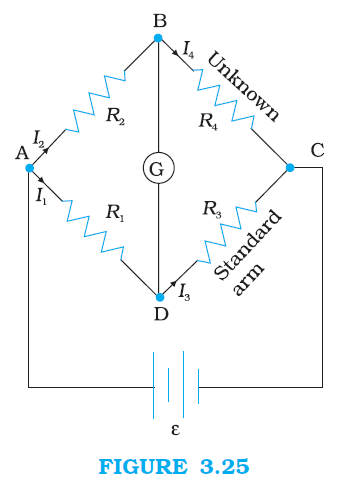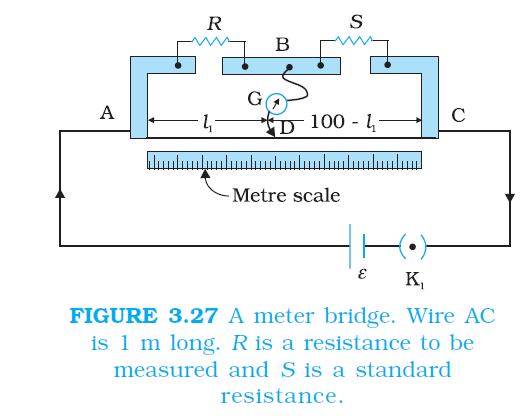Physics WHEATSTONE BRIDGE ,METER BRIDGE, FOR CBSE-NCERT-6

### Topic covered

color{blue}{star} WHEATSTONE BRIDGE
color{blue}{star} METER BRIDGE
color{blue}{star} POTENTIOMETER

### WHEATSTONE BRIDGEcolor{blue} ✍️ As an application of Kirchhoff’s rules consider the circuit shown in Fig. 3.25, which is called the Wheatstone bridge.

color{blue} ✍️ The bridge has four resistors color{blue}(R_1, R_2, R_3) and R_4. Across one pair of diagonally opposite points (A and C in the figure) a source is connected. This (i.e., AC) is called the battery arm.

color{blue} ✍️ Between the other two vertices, B and D, a galvanometer G (which is a device to detect currents) is connected. This line, shown as BD in the figure, is called the galvanometer arm.

color{blue} ✍️ For simplicity, we assume that the cell has no internal resistance. In general there will be currents flowing across all the resistors as well as a current I_g through G. Of special interest, is the case of a balanced bridge where the resistors are such that I_g = 0.

color{blue} ✍️ We can easily get the balance condition, such that there is no current through G. In this case, the Kirchhoff’s junction rule applied to junctions D and  B (see the figure) immediately gives us the relations color{blue}(I_1 = I_3) and color{blue}(I_2 = I_4).

color{blue} ✍️ Next, we apply Kirchhoff’s loop rule to closed loops ADBA and CBDC. The first loop gives

color{blue}(–I_1 R_1 + 0 + I_2 R_2 = 0 \ \ \ \ (Ig = 0))

.........(3.81)

=> and the second loop gives, upon using color{blue}(I_3 = I_1, I_4 = I_2)

color{blue}(I_2 R_4 + 0 – I_1 R_3 = 0)

...........(3.82)

From Eq. (3.81), we obtain, color{blue}((I_1)/(I_2) = (R_2)/(R_1))

whereas from Eq. (3.82), we obtain, color{blue}((I_1)/(I_2) = (R_4)/(R_3))

Hence, we obtain the condition

color{blue}((R_2)/(R_1) = (R_4)/(R_3))

...........[3.83(a)]

color{blue} ✍️ This last equation relating the four resistors is called the balance condition for the galvanometer to give zero or null deflection.

color{blue} ✍️ The Wheatstone bridge and its balance condition provide a practical method for determination of an unknown resistance.

color{blue} ✍️ Let us suppose we have an unknown resistance, which we insert in the fourth arm; R_4 is thus not known. Keeping known resistances R_1 and R_2 in the first and second arm of the bridge, we go on varying R_3 till the galvanometer shows a null deflection.

color{blue} ✍️ The bridge then is balanced, and from the balance condition the value of the unknown resistance R_4 is given by

color{blue}(R_4 = R_3 (R_2)/(R_1))

.........[3.83(b)]

color{blue} ✍️ A practical device using this principle is called the meter bridge. It will be discussed in the next section.
Q 3174191956The four arms of a Wheatstone bridge (Fig. 3.26) have the following resistances:
AB = 100Ω, BC = 10Ω, CD = 5Ω, and DA = 60Ω.
A galvanometer of 15Ω resistance is connected across BD. Calculate the current through the galvanometer when a potential difference of 10 V is maintained across AC.
Class 12 Chapter example 8Solution:

Considering the mesh BADB, we have
100I_1 + 15I_g – 60I_2 = 0

or 20I_1 + 3I_g – 12I_2= 0

Considering the mesh BCDB, we have

10 (I_1 – I_g) – 15I_g – 5 (I_2 + I_g) = 0
10I_1 – 30I_g –5I_2 = 0
2I_1 – 6I_g – I_2 = 0

Considering the mesh ADCEA,

60I_2 + 5 (I_2 + I_g) = 10

65I_2 + 5I_g = 10

13I_2 + I_g = 2
Multiplying Eq. (3.84b) by 10

20I_1 – 60I_g – 10I_2 = 0

From Eqs. (3.84d) and (3.84a) we have

63I_g – 2I_2 = 0
I_2 = 31.5I_g

Substituting the value of I_2 into Eq. [3.84(c)], we get
13 (31.5I_g ) + I_g = 2
410.5 I_g = 2
I_g = 4.87 mA.

### METER BRIDGEcolor{blue} ✍️ The meter bridge is shown in Fig. 3.27. It consists of a wire of length 1m and of uniform cross sectional area stretched taut and clamped between two thick metallic strips bent at right angles, as shown.

color{blue} ✍️ The metallic strip has two gaps across which resistors can be connected. The end points where the wire is clamped are connected to a cell through a key. One end of a galvanometer is connected to the metallic strip midway between the two gaps.

color{blue} ✍️ The other end of the galvanometer is connected to a ‘jockey’. The jockey is essentially a metallic rod whose one end has a knife-edge which can slide over the wire to make electrical connection.

color{blue} ✍️ R is an unknown resistance whose value we want to determine. It is connected across one of the gaps. Across the other gap, we connect a standard known resistance S. The jockey is connected to some point D on the wire, a distance l cm from the end A.

color{blue} ✍️ The jockey can be moved along the wire. The portion AD of the wire has a resistance R_(cm)l, where R_(cm) is the resistance of the wire per unit centimetre.

color{blue} ✍️ The portion DC of the wire similarly has a resistance color{blue}(R_(cm) (100-l)). The four arms AB, BC, DA and CD [with resistances R, S, R_(cm) l and color{blue}(R_(cm)(100-l)) obviously form a Wheatstone bridge with AC as the battery arm and BD the galvanometer arm.

color{blue} ✍️ If the jockey is moved along the wire, then there will be one position where the galvanometer will show no current.

color{blue} ✍️ Let the distance of the jockey from the end A at the balance point be l= l_1. The four resistances of the bridge at the balance point then are color{blue}(R, S, R_(cm) l_1) and color{blue}(R_(cm)(100–l_1).) The balance condition, Eq. [3.83(a)] gives

color{blue}(R/S = (R_(cm)l_1)/(R_(cm)(100-l_1)) = (l_1)/(100-l_1))

.........(3.85)

color{blue} ✍️ Thus, once we have found out l_1, the unknown resistance R is known in terms of the standard known resistance S by

color{blue}(R = S (l_1)/(100 - l_1))

..........(3.86)

color{blue} ✍️ By choosing various values of S, we would get various values of l_1, and calculate R each time. An error in measurement of l_1 would naturally result in an error in R. It can be shown that the percentage error in R can be minimised by adjusting the balance point near the middle of the bridge, i.e., when l_1 is close to 50 cm. (This requires a suitable choice of S.)
Q 3115101060In a metre bridge (Fig. 3.27), the null point is found at a distance of 33.7 cm from A. If now a resistance of 12Ω is connected in parallel with
Class 12 Chapter example 9Solution:

From the first balance point, we get

R/S = (33.7)/(66.3)

After S is connected in parallel with a resistance of 12Ω , the resistance across the gap changes from S to S_(eq,) where

S_(sq) = (12S)/(S+12)

and hence the new balance condition now gives

(51.9)/(48.1) = R/S_(Sq) = R((S+12)/(12S))

Substituting the value of R/S from Eq. (3.87), we get

(51.9)/(48.1) = (S+12)/(12) • (33.7)/(66.3)

which gives S = 13.5Ω. Using the value of R/S above, we get R = 6.86 Ω.

### POTENTIOMETER

color{blue} ✍️ This is a versatile instrument. It is basically a long piece of uniform wire, sometimes a few meters in length across which a standard cell is connected.

color{blue} ✍️ In actual design, the wire is sometimes cut in several pieces placed side by side and connected at the ends by thick metal strip. (Fig. 3.28). In the figure, the wires run from A to C.color{blue} ✍️ The small vertical portions are the thick metal strips connecting the various sections of the wire. A current I flows through the wire which can be varied by a variable resistance (rheostat, R) in the circuit. Since the wire is uniform, the potential difference between A and any point at a distance l from A is

epsilon(l) = phil

..........(3.89)

color {blue}{➢➢} where φ is the potential drop per unit length. Figure 3.28 (a) shows an application of the potentiometer to compare the emf of two cells of emf ε_1 and ε_2 .

color{blue} ✍️ The points marked 1, 2, 3 form a two way key. Consider first a position of the key where 1 and 3 are connected so that the galvanometer is connected to ε_1.

color{blue} ✍️ The jockey is moved along the wire till at a point N_1, at a distance l_1 from A, there is no deflection in the galvanometer. We can apply Kirchhoff’s loop rule to the closed loop color{blue}(AN_1G31A) and get,

color{blue}(phi l_1 + 0 - epsilon_1 =0)

...........(3.90)

color {blue}{➢➢}Similarly, if another emf ε_2 is balanced against color{blue}(l_2 (AN_2))

color{blue}(phi l_2 + 0 - epsilon_2=0)

.........(3.91)

From the last two equations

color{blue}((epsilon_1)/(epsilon_2) = (l_1)/(l_2))

..........(3.92)

color{blue} ✍️ This simple mechanism thus allows one to compare the emf’s of any two sources.

color{blue} ✍️ In practice one of the cells is chosen as a standard cell whose emf is known to a high degree of accuracy. The emf of the other cell is then easily calculated from Eq. (3.92). We can also use a potentiometer to measure internal resistance of a cell [Fig. 3.28 (b)].

color{blue} ✍️ For this the cell (emf ε ) whose internal resistance (r) is to be determined is connected across a resistance box through a key K_2, as shown in the figure. With key K_2 open, balance is obtained at length l_1 (AN_1). Then,

color{blue}(epsilon= phil_1)

.....[3.93(a)]

color{blue} ✍️ When key K_2 is closed, the cell sends a current (I ) through the resistance box (R). If V is the terminal potential difference of the cell and balance is obtained at length color{blue}(L_2 (AN_2)),

color{blue}(V = phil_2)

...........[3.93(b)]

color{blue} ✍️ So we have

color{blue}(ε//V= l_1//l_2)

............[3.94(a)]

But, color{blue}(ε = I (r + R)) and color{blue}(V = IR). This gives

color{blue}(ε//V = (r+R)//R)

...........[3.94(b)]
From Eq. [3.94(a)] and [3.94(b)] we have

color{blue}((R+r)//R = l_1//l_2)

color{blue}(r = R (l_1/l_2-1)

..............(3.95)

color{blue} ✍️ Using Eq. (3.95) we can find the internal resistance of a given cell. The potentiometer has the advantage that it draws no current from the voltage source being measured. As such it is unaffected by the internal resistance of the source
Q 3155101064A resistance of R Ω draws current from a potentiometer. The potentiometer has a total resistance R_0 Ω (Fig. 3.29). A voltage V is supplied to the potentiometer. Derive an expression for the voltage across R when the sliding contact is in the middle of the potentiometer.
Class 12 Chapter example 10Solution:

While the slide is in the middle of the potentiometer only half of its resistance (R_0//2) will be between the points A and B. Hence, the total resistance between A and B, say, R_1, will be given by the following expression:

(1)/(R_1) = 1/R + (1)/(R_0/2)

R_1 = (R_0R)/(R_0+2R)

The total resistance between A and C will be sum of resistance between A and B and B and C, i.e., R_1 + R_0//2

∴ The current flowing through the potentiometer will be

I = (V)/(R_1+R_0//2) = (2V)/(2R_1+R_0)

The voltage V_1 taken from the potentiometer will be the product of
current I and resistance R_1,

V_1 = IR_I = ((2V)/(2R_1+R_0))xxR_1

Substituting for R_1, we have a

V_1 =((2V)/((R_0xxR)/(R_0+2R))) xx (R_0xxR)/(R_0+2R)

V_1 = (2VR)/(2R+R_0+2R)

or V_1 = (2VR)/(R_0+4R)